# Lab 18.2 Varrays

 Team-Fly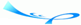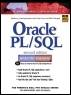Oracle® PL/SQL® Interactive Workbook, Second EditionBy Benjamin Rosenzweig, Elena Silvestrova Table of Contents Chapter 18.  Collections

Lab Objective

After this Lab, you will be able to:

• Use Varrays

As mentioned earlier, a varray is another collection type, and it stands for variable-size arrays. Similar to PL/SQL tables, each element of a varray is assigned a consecutive subscript starting at 1, as shown in Figure 18.2.

##### Figure 18.2. Varray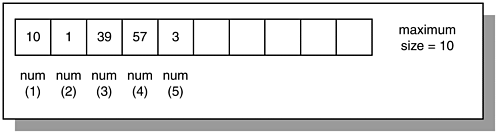Figure 18.2 shows a varray consisting of five integer numbers. Each number is assigned a unique subscript that corresponds to its position in the varray.

It is important to note that a varray has a maximum size. In other words, a subscript of a varray has a fixed lower bound equal to 1, and an upper bound that is extensible if such a need arises. In Figure 18.2, the upper bound of a varray is 5, but it can be extended to 6, 7, 8, and so on up to 10. Therefore, a varray can contain a number of elements, varying from zero (empty array) to its maximum size. You will recall that PL/SQL tables do not have a maximum size that must be specified explicitly.

The general syntax for creating a varray is as follows (the reserved words and phrases surrounded by brackets are optional):

` TYPE type_name IS {VARRAY | VARYING ARRAY} (size_limit) OF  element_type [NOT NULL];  varray_name TYPE_NAME; `

First, a varray structure is defined using the TYPE statement, where TYPE_NAME is the name of the type that is used in the second step to declare an actual varray. Notice that there are two variations of the type, VARRAY and VARYING ARRAY. A SIZE_LIMIT is a positive integer literal that specifies the upper bound of a varray. Just like in the case of PL/SQL tables, there are restrictions that apply to an ELEMENT_TYPE of a varray. These restrictions are listed in Oracle help available online. Second, the actual varray is declared based on the type specified in the first step.

Consider the following code fragment: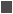FOR EXAMPLE

` DECLARE     TYPE last_name_type IS VARRAY(10) OF student.     last_name%TYPE;     last_name_varray last_name_type; `

In this example, type last_name_type is declared as a varray of ten elements based on the column LAST_NAME of the STUDENT table. Next, the actual varray last_name_varray is declared based on the LAST_NAME_TYPE.

Similar to nested tables, a varray is automatically NULL when it is declared and must be initialized before its individual elements can be referenced. So consider a modified version of the example used in the previous lab. Instead of using nested table, this version uses varray (changes are highlighted in bold).FOR EXAMPLE

` DECLARE     CURSOR name_cur IS        SELECT last_name          FROM student         WHERE rownum <= 10;     TYPE last_name_type IS VARRAY(10) OF student.     last_name%TYPE;     last_name_varray last_name_type := last_name_type();     v_counter INTEGER := 0;  BEGIN     FOR name_rec IN name_cur LOOP        v_counter := v_counter + 1;        last_name_varray.EXTEND;        last_name_varray(v_counter) := name_rec.last_name;        DBMS_OUTPUT.PUT_LINE ('last_name('||v_counter||'): '||           last_name_varray(v_counter));     END LOOP;  END; `

This example produces the following output:

` last_name(1): Crocitto  last_name(2): Landry  last_name(3): Enison  last_name(4): Moskowitz  last_name(5): Olvsade  last_name(6): Mierzwa  last_name(7): Sethi  last_name(8): Walter  last_name(9): Martin  last_name(10): Noviello  PL/SQL procedure successfully completed. `

Based on the preceding example, you may realize that collection methods seen in the previous lab can be used with varrays as well. Consider the following example, which illustrates the use of various collection methods when applied to a varray.FOR EXAMPLE

` DECLARE     TYPE varray_type IS VARRAY(10) OF NUMBER;     varray varray_type := varray_type(1, 2, 3, 4, 5, 6);  BEGIN     DBMS_OUTPUT.PUT_LINE ('varray.COUNT = '||varray.COUNT);     DBMS_OUTPUT.PUT_LINE ('varray.LIMIT = '||varray.LIMIT);     DBMS_OUTPUT.PUT_LINE ('varray.FIRST = '||varray.FIRST);     DBMS_OUTPUT.PUT_LINE ('varray.LAST = '||varray.LAST);     varray.EXTEND(2, 4);     DBMS_OUTPUT.PUT_LINE ('varray.LAST = '||varray.LAST);     DBMS_OUTPUT.PUT_LINE ('varray('||varray.LAST||') = '||        varray(varray.LAST));     -- Trim last two elements     varray.TRIM(2);     DBMS_OUTPUT.PUT_LINE('varray.LAST = '||varray.LAST);  END; `

Consider the output returned by the example:

` varray.COUNT = 6  varray.LIMIT = 10  varray.FIRST = 1  varray.LAST = 6  varray.LAST = 8  varray(8) = 4  varray.LAST = 6  PL/SQL procedure successfully completed. `

The first two lines of output

` varray.COUNT = 6  varray.LIMIT = 10 `

show the results of the COUNT and LIMIT methods, respectively. You will recall that the COUNT method returns the number of elements that a collection contains. The collection has been initialized to six elements, so the COUNT method returns a value of 6.

The next line of output corresponds to another collection method, LIMIT. This method returns the maximum number of elements that a collection can contain and is usually used with varrays only because varrays have an upper bound specified at the time of declaration. The collection VARRAY has an upper bound of ten, so the LIMIT method returns a value of 10. When used with nested tables, the LIMIT method returns NULL because nested tables do not have a maximum size.

The third and fourth lines of the output

` varray.FIRST = 1  varray.LAST = 6 `

show the results of the FIRST and LAST methods.

The fifth and six lines of the output

` varray.LAST = 8  varray(8) = 4 `

show the results of LAST method and the value of the eighth element of the collection after the EXTEND method increased the size of the collection. Notice that the EXTEND method

` varray.EXTEND(2, 4); `

appends two copies on the fourth element to the collection. As a result, the seventh and eighth elements both contain a value of 4.

Next, the last line of output

` varray.LAST = 6 `

shows the value of the last subscript after the last two elements were removed via the TRIM method.It is important to note that you cannot use the DELETE method with a varray to remove its elements. Unlike PL/SQL tables, varrays are dense, and using the DELETE method causes an error, as illustrated in the following example:

` DECLARE     TYPE varray_type IS VARRAY(3) OF CHAR(1);     varray varray_type := varray_type('A', 'B', 'C');  BEGIN     varray.DELETE(3);  END;     varray.DELETE(3);     *  ERROR at line 6:  ORA-06550: line 6, column 4:  PLS-00306: wrong number or types of arguments in call to  'DELETE'  ORA-06550: line 6, column 4:  PL/SQL: Statement ignored `

#### Lab 18.2 Exercises

##### 18.2.1 Use Varrays

In this exercise, you will learn more about varrays. You will need to debug the following script, which populates city_varray with 10 cities selected from the ZIPCODE table and displays its individual elements on the screen.

Create the following PL/SQL script:

` -- ch18_3a.sql, version 1.0  SET SERVEROUTPUT ON  DECLARE     CURSOR city_cur IS        SELECT city          FROM zipcode         WHERE rownum <= 10;     TYPE city_type IS VARRAY(10) OF zipcode.city%TYPE;     city_varray city_type;     v_counter INTEGER := 0;  BEGIN     FOR city_rec IN city_cur LOOP        v_counter := v_counter + 1;        city_varray(v_counter) := city_rec.city;        DBMS_OUTPUT.PUT_LINE('city_varray('||v_counter||           '): '||city_varray(v_counter));     END LOOP;  END; `

Execute the script, and then answer the following questions:

 a) What output was printed on the screen? Explain it. b) Modify the script so that no errors are returned at runtime. c) Modify the script as follows: Double the size of the varray and populate the last ten elements with the first ten elements. In other words, the value of the eleventh element should be equal to the value of the first element; the value of the twelfth element should be equal to the value of the second element; and so forth.

#### Lab 18.2 Exercise Answers

This section gives you some suggested answers to the questions in Lab 18.2, with discussion related to how those answers resulted. The most important thing to realize is whether your answer works. You should figure out the implications of the answers here and what the effects are from any different answers you may come up with.

##### 18.2.1 Answers

 a) What output was printed on the screen? Explain it. A1: Answer: Your output should look similar to the following:` DECLARE * ERROR at line 1: ORA-06531: Reference to uninitialized collection ORA-06512: at line 14 `You will recall that when a varray is declared, it is automatically NULL. In other words, the collection itself is NULL, not its individual elements. Therefore, before it can be used, it must be initialized via the constructor function with the same name as the varray type. Furthermore, once the collection is initialized, the EXTEND method must be used before its individual elements can be referenced in the script. b) Modify the script so that no errors are returned at runtime. A2: Answer: Your script should look similar to the following script. Changes are shown in bold letters.` -- ch18_3b.sql, version 2.0 SET SERVEROUTPUT ON DECLARE CURSOR city_cur IS SELECT city FROM zipcode WHERE rownum <= 10; TYPE city_type IS VARRAY(10) OF zipcode.city%TYPE; city_varray city_type := city_type(); v_counter INTEGER := 0; BEGIN FOR city_rec IN city_cur LOOP v_counter := v_counter + 1; city_varray.EXTEND; city_varray(v_counter) := city_rec.city; DBMS_OUTPUT.PUT_LINE('city_varray('||v_counter|| '): '||city_varray(v_counter)); END LOOP; END; `When run, this script produces the following output:` city_varray(1): Santurce city_varray(2): North Adams city_varray(3): Dorchester city_varray(4): Tufts Univ. Bedford city_varray(5): Weymouth city_varray(6): Sandwich city_varray(7): Ansonia city_varray(8): Middlefield city_varray(9): Oxford city_varray(10): New Haven PL/SQL procedure successfully completed. ` c) Modify the script as follows: Double the size of the varray and populate the last ten elements with the first ten elements. In other words, the value of the eleventh element should be equal to the value of the first element; the value of the twelfth element should be equal to the value of the second element; and so forth. A3: Answer: Your script should look similar to the following script. Changes are shown in bold letters.` -- ch18_3c.sql, version 3.0 SET SERVEROUTPUT ON DECLARE CURSOR city_cur IS SELECT city FROM zipcode WHERE rownum <= 10; TYPE city_type IS VARRAY(20) OF zipcode.city%TYPE; city_varray city_type := city_type(); v_counter INTEGER := 0; BEGIN FOR city_rec IN city_cur LOOP v_counter := v_counter + 1; city_varray.EXTEND; city_varray(v_counter) := city_rec.city; END LOOP; FOR i IN 1..v_counter LOOP -- extend the size of varray by 1 and copy the -- current element to the last element city_varray.EXTEND(1, i); END LOOP; FOR i IN 1..20 LOOP DBMS_OUTPUT.PUT_LINE('city_varray('||i|| '): '||city_varray(i)); END LOOP; END; `In the preceding script, you increase the maximum size of the varray to 20 at the time of city_type declaration. After the first 10 elements of the varray are populated, the last ten elements are populated via numeric FOR loop and the EXTEND method as follows:` FOR i IN 1..v_counter LOOP -- extend the size of varray by 1 and copy the current -- element to the last element city_varray.EXTEND(1, i); END LOOP; `In this loop, the loop counter is implicitly incremented by one. So for the first iteration of the loop, the size of the varray is increased by one and the first element of the varray is copied to the eleventh element. In the same manner, the second element of the varray is copied to the twelfth element, and so forth.In order to display all elements of the varray, the DBMS_OUTPUT.PUT_LINE statement has been moved to its own numeric FOR loop that iterates 20 times.When run, this script produces the following output:` city_varray(1): Santurce city_varray(2): North Adams city_varray(3): Dorchester city_varray(4): Tufts Univ. Bedford city_varray(5): Weymouth city_varray(6): Sandwich city_varray(7): Ansonia city_varray(8): Middlefield city_varray(9): Oxford city_varray(10): New Haven city_varray(11): Santurce city_varray(12): North Adams city_varray(13): Dorchester city_varray(14): Tufts Univ. Bedford city_varray(15): Weymouth city_varray(16): Sandwich city_varray(17): Ansonia city_varray(18): Middlefield city_varray(19): Oxford city_varray(20): New Haven PL/SQL procedure successfully completed. `

#### Lab 18.2 Self-Review Questions

In order to test your progress, you should be able to answer the following questions.

Answers appear in Appendix A, Section 18.2.

 1) One of the differences between the nested tables and varrays is that _____ nested tables can be sparse, and varrays cannot._____ varrays can be sparse, and nested tables cannot. 2) A varray has an upper bound that _____ can be extended without any limits._____ can be extended to its maximum size._____ is fixed and cannot be extended to all. 3) A varray must be initialized prior to its use. _____ True_____ False 4) If a varray has maximum size of 5 and contains 2 elements _____ the LIMIT and COUNT methods return the same value of 5._____ the LIMIT and COUNT methods return the same value of 2._____ the LIMIT method returns the value of 5, and the COUNT method returns the value of 2. 5) If a varray has eight elements, the DELETE (3, 7) method _____ deletes the third and seventh elements of the collection._____ deletes the third element and ignores the seventh element of the collection._____ deletes the elements three to seven._____ causes an error.

 Team-FlyTop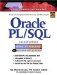Oracle PL/SQL Interactive Workbook (2nd Edition)
ISBN: 0130473200
EAN: 2147483647
Year: 2002
Pages: 146

Similar book on Amazon

flylib.com © 2008-2017.
If you may any questions please contact us: flylib@qtcs.net